# 330 Christmas Factor Trees

### Today’s Puzzle:

Can you find the factors and complete this Christmas tree multiplication table?Print the puzzles or type the factors on this excel file:  10 Factors 2014-12-22

### Factor Trees for 330:

Within these seven factor trees for 330 there are also factor trees for 6, 10, 15, 22, 30, 33, 55, 66, 110, and 165, the tops of which are all in brown. The prime factors of 330 are all in red.### Factors of 330:

• 330 is a composite number.
• Prime factorization: 330 = 2 x 3 x 5 x 11
• The exponents in the prime factorization are 1, 1, 1, and 1. Adding one to each and multiplying we get (1 + 1)(1 + 1)(1 + 1)(1 + 1) = 2 x 2 x 2 x 2 = 16. Therefore 330 has exactly 16 factors.
• Factors of 330: 1, 2, 3, 5, 6, 10, 11, 15, 22, 30, 33, 55, 66, 110, 165, 330
• Factor pairs: 330 = 1 x 330, 2 x 165, 3 x 110, 5 x 66, 6 x 55, 10 x 33, 11 x 30, or 15 x 22
• 330 has no square factors that allow its square root to be simplified. √330 ≈ 18.166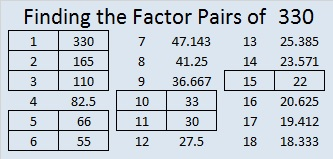### Sum-Difference Puzzle:

330 has eight factor pairs. The numbers in one of those pairs add up to 61, and the numbers in another one subtract to 61. If you can identify those factors, then you can solve this puzzle!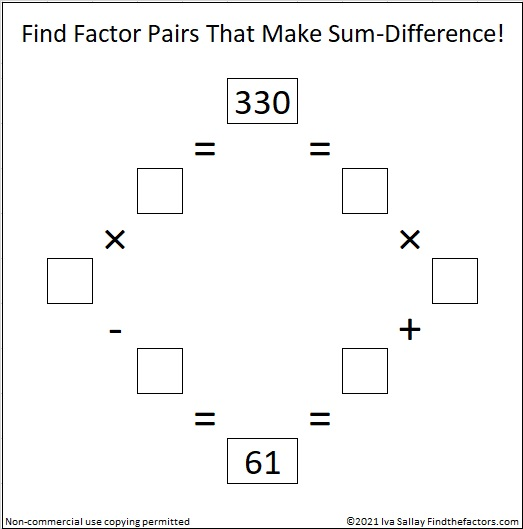### Tree Puzzle Solution: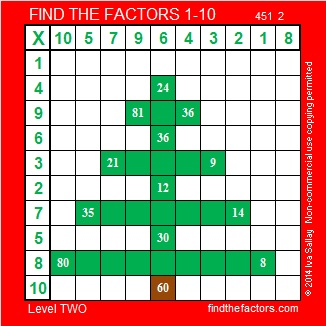# 17 Christmas Angels

• 17 is a prime number.
• Prime factorization: 17 is prime.
• The exponent of prime number 17 is 1. Adding 1 to that exponent we get (1 + 1) = 2. Therefore 17 has exactly 2 factors.
• Factors of 17: 1, 17
• Factor pairs: 17 = 1 x 17
• 17 has no square factors that allow its square root to be simplified. √17 ≈ 4.123.How do we know that 17 is a prime number? If 17 were not a prime number, then it would be divisible by at least one prime number less than or equal to √17 ≈ 4.1. Since 17 cannot be divided evenly by 2 or 3, we know that 17 is a prime number.

17 is never a clue in the FIND THE FACTORS puzzles.

Many Christmas trees in the United States have been up and decorated for weeks. Some of them have a beautiful angel on the top to remind us of the angel that visited the shepherds. In Hungary, the angel is remembered in a different way. There the Christmas tree is put up on Christmas Eve. Tradition says that angels are the ones who decorate the tree with the delicious candies called szaloncukor. The candies are wrapped in specially prepared white tissue and fastened to the tree with white yarn. See the related articles at the end of the post for more information about this fascinating tradition.

The angel puzzles that I’ve made for this post have a few extra clues so they will be easier to solve. The first level 5 puzzle even has many of the same clues as the level 4 puzzle. Nevertheless, be careful because each level 5 angel has a few tricks up her sleeve. Still if you can write the numbers 1 to 12 in both the top row and the first column so that those numbers are the factors of the given clues, then you’ve solved the puzzle. There is only one solution to each puzzle. Click 12 Factors 2013-12-19 for a printable version of these and a few other puzzles.

Hungary:

United States:

# 15 is the Magic Sum of a 3 x 3 Magic Square

15 is a composite number. 15 = 1 x 15 or 3 x 5. Factors of 15: 1, 3, 5, 15. Prime factorization: 15 = 3 x 5.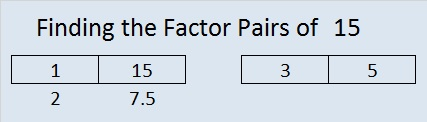When 15 is a clue in the FIND THE FACTORS 1 – 10 or 1 – 12 puzzles, use 3 and 5 as the factors.

If you added the first nine counting numbers together, what sum would you get? What is 1 + 2 +3 + 4+ 5 + 6 + 7 + 8 + 9?

Would you get the same answer by adding (1 + 9) + (2 + 8) + (3 +7) + (4 + 6) + 5?

These are two of the many fun questions you can explore when you try to make a magic square. What is a magic square? If you can place the numbers from 1 to 9 in the box below so that the sum of any row, column, or diagonal will equal the sum of any other row, column, or diagonal, then you will have made a 3 x 3 magic square. The sum of a row, column, or diagonal in a magic square is called the magic sum.Clearly it is not a magic square yet. In fact, only one of the numbers is positioned where it needs to be. Which number do you think is already in the correct position?

When it becomes a magic square, what will the magic sum be? One student noticed that in its current state the sums of the rows are 6, 15, and 24. The sums of the columns are 12, 15, 18. The sums of the diagonals are 15 and 15. Since 15 occurs most often, could the magic sum be 15? One way to determine what the magic sum should be is to add the sums of all three rows and then divide by the number of rows. Since 1 + 2 + 3 + 4 + 5 + 6 + 7 + 8 + 9 = 45 and 45 ÷ 3 = 15, then 15 is indeed the magic sum.

Here are a few easy-to-remember steps to construct a 3 x 3 magic square quickly.

Step 1: Draw a tic-tac-toe board and put 5 in the middle.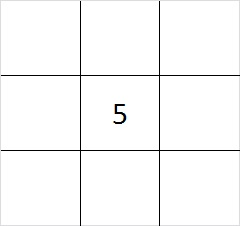Step 2: Put one of the even numbers in one of the corners.  You have four different choices, 2, 4, 6, or 8. The illustration is for the number 2, but any of the even numbers will work.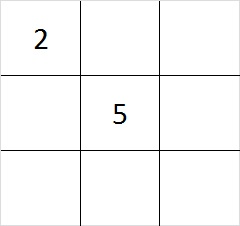Step 3: Subtract your even number from 10 to find its partner. 4 + 6 are partners and so are 2 + 8. Put the partner of the number you chose for step 1 in the corner that is diagonal to it.Step 4: Put the other two even numbers in the remaining corners. Yes, you have two choices where to put the numbers. Either choice will work.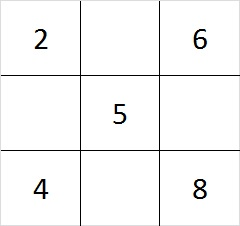Step 5: Since 6 + 8 = 14 and 15 – 14 = 1, put 1 in the cell between the 6 and the 8. Do similar addition and subtraction problems on each side of the square to determine where to place the 3, 7, and 9. You can work clockwise or counter clockwise, or skip around the square doing the addition and subtraction problems; it doesn’t matter.

This finished magic square looks like this: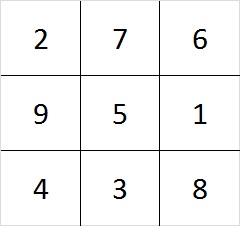Check it out! Every row, column, and diagonal adds up to 15!

As we created the square, we made choices. First we chose between 4 even numbers, and later we had 2 more choices. Notice that 4 x 2 = 8. There are 8 different ways to make a 3 x 3 magic square! (However, they are all really the same square turned upside down, rolled on its side, viewed from the back. etc.)

There are 880 different ways to make a 4 x 4 magic square. Look over the related articles at the end of this post to learn more about magic squares that are bigger than 3 x 3.

Speaking of magic squares, when I look at the square logic puzzle below, something magical happens. This puzzle has nine clues in it, and all of them are perfect squares. I can use those nine clues to construct a complete multiplication table. If you finish the same puzzle, your multiplication table will look exactly like mine because this puzzle has only one solution.The level 3 puzzle below is only a little bit more difficult. To solve it place the numbers 1 – 10 in the top row and again in the first column so that those placed numbers are the factors of the given clues. Again there is only one solution, and you will need to use logic to find it. Click 10 Factors 2014-01-06 for more puzzles and last week’s answers.May we all find a little bit more magic in our lives!

# 14 Oh Christmas Tree

14 is a composite number. 14 = 1 x 14 or 2 x 7. Factors of 14: 1, 2, 7, 14. Prime factorization: 14 = 2 x 7.When 14 is a clue in the FIND THE FACTORS  1 – 10 or 1 – 12 puzzles, use 2 and 7 as the factors.

O Christmas Tree, O Christmas Tree,